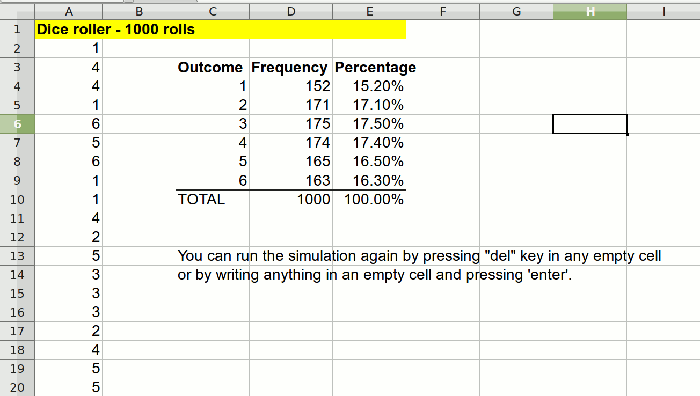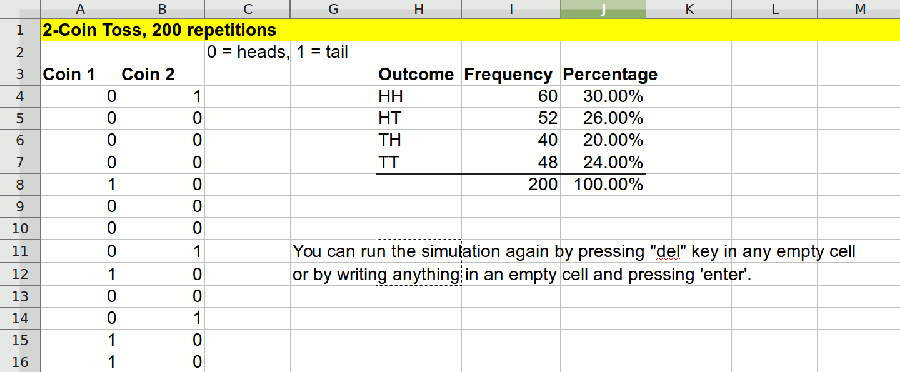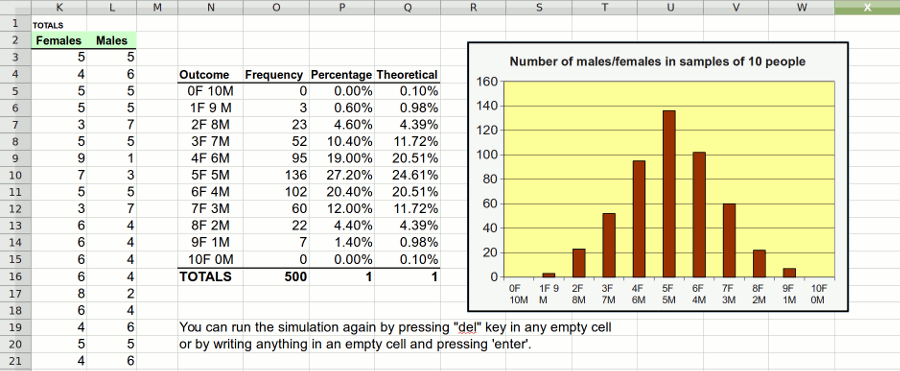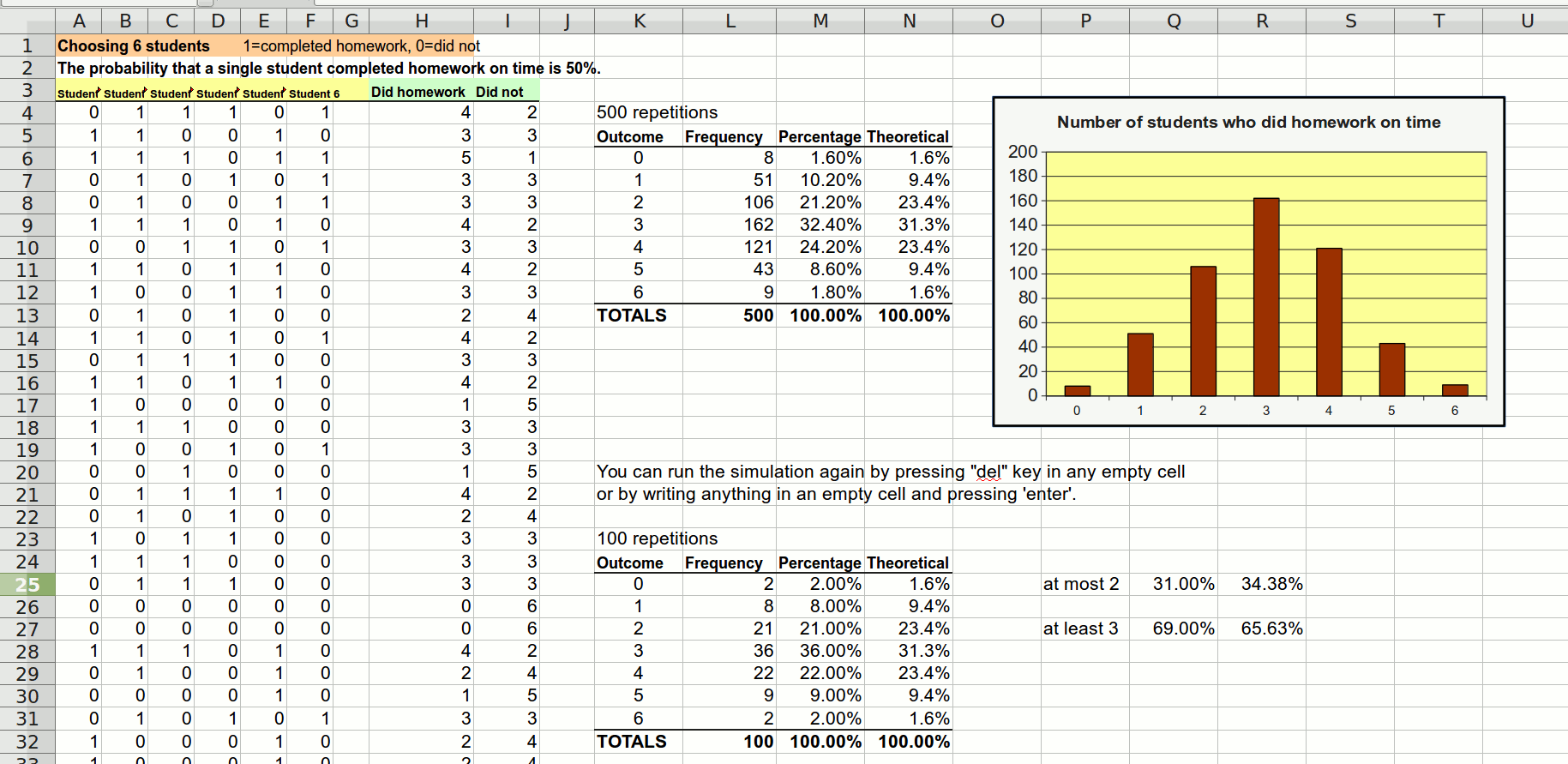^
You are here: HomeLessons → Probability simulations

# Free probability simulations for 7th grade

These interactive probability simulations work in MS Excel, LibreOffice Calc, or any other spreadsheet program that can open xls files. Feel free to download them and use them with your students.

They use random digits and are designed for the probability lessons in Math Mammoth Grade 7 curriculum, but you can also use them without it.

## 1. Dice rollerThis is a simple virtual die roller (one die). It includes different sheets for 500 rolls, 1000 rolls, and 2000 rolls, so you can compare the three, and see how the experimental probabilities gets closer to the theoretical ones as we increase the number of rolls. The spreadsheet automatically calculates the frequencies for all six outcomes (1, 2, 3, 4, 5, 6) and their experimental probabilities.

Click to download or open Dice-roller.xls (Excel file).

## 2. Two-coin tossThis simulation uses 1s and 0s to represent heads and tails of two coins. It contains two sheets: one for 200 tosses and the other for 500 tosses. The spreadsheet automatically calculates the frequencies for all four outcomes (HH, HT, TH, TT) and their experimental probabilities.

Click to download or open two-coin-toss.xls (Excel file).

## 3. Number of males and females in a sample of 10 people

If you choose 10 people randomly, what is the probability that exactly 5 of them are male and 5 are female? Or that exactly 7 or them are male and 3 female? This simulation explores the situation with either 100, 500, or 2000 repetitions (or samples).

Click to download or open 10-people-females-males.xls (Excel file).## 4. Sample of six students

This is a more complex simulation. We choose a sample of six students from a student population where the probability that a single student completed homework on time is 50%. The sampling is repeated either 100 or 500 times. We observe how many students out of the six completed homework on time.

The simulation uses random digits so that 0 represents a student who didn't complete homework on time and 1 represents a student who did. The spreadsheet automatically calculates the frequencies of each outcome (0-6 students), the probabilities, and draws a bar graph for the distribution.

The second sheet in the spreadsheet explores the same situation, but this time the probability that a single student completed homework on time is 70%.

Click to download or open random-digits-six-students.xls (Excel file).Probability video lessons by Maria

Math Mammoth Statistics & Probability — worktext for grades 6-7

Math Mammoth Grade 7 curriculum

By Maria Miller

WAIT!

Receive my monthly collection of math tips & resources directly in your inbox — and get a FREE Math Mammoth book!We respect your email privacy.
You can unsubscribe at any time.

### Math Mammoth Tour

Confused about the different options? Take a virtual email tour around Math Mammoth! You'll receive:

An initial email to download your GIFT of over 400 free worksheets and sample pages from my books. Six other "TOURSTOP" emails that explain the important things and commonly asked questions concerning Math Mammoth curriculum. (Find out the differences between all these different-colored series!)

This way, you'll have time to digest the information over one or two weeks, plus an opportunity to ask me personally about the curriculum.
A monthly collection of math teaching tips & Math Mammoth updates (unsubscribe any time)We respect your email privacy.

Note: You will FIRST get an email that asks you to confirm your email address. If you cannot find this confirmation email, please check your SPAM/JUNK folder.

### "Mini" Math Teaching Course

This is a little "virtual" 2-week course, where you will receive emails on important topics on teaching math, including:

- How to help a student who is behind
- Troubles with word problems
- Teaching multiplication tables
- Why fractions are so difficult
- The value of mistakes
- Should you use timed tests
- And more!

You will also receive:

A GIFT of over 400 free worksheets and sample pages from my books right in the very beginning.A monthly collection of math teaching tips & Math Mammoth updates (unsubscribe any time)
We respect your email privacy.

Note: You will FIRST get an email that asks you to confirm your email address. If you cannot find this confirmation email, please check your SPAM/JUNK folder.

### Maria's Math Tips

Enter your email to receive math teaching tips, resources, Math Mammoth news & sales, humor, and more! I tend to send out these tips about once monthly, near the beginning of the month, but occasionally you may hear from me twice per month (and sometimes less often).You will also receive:

• A GIFT of over 400 free worksheets and sample pages from my books.We respect your email privacy.